# Which functions support "Formula" in the summary method.Hello,

Is there a list somewhere that shows which functions support "Formula" in the summary method?

Tagged:

•For this particular scenario, since you need to calculate things differently at different levels of the time hierarchy, I would recommend exploring the Ratio Summary Method. Example created below:

I have started by creating different lines for each time summary with its relevant formula in it.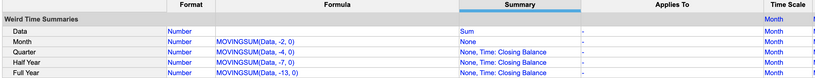Notice the summary here is showing as "Time: Closing Balance". I am calculating the data at the month level, and showing it in the summaries properly.

Since we have it in multiple lines, now we need to combine it.

Adding to that same module, I have line items that are getting the data to the actual level that we want it at, The year, Half-year, quarter, and month. This is essentially staging the data out so that we can use the ratio summary method.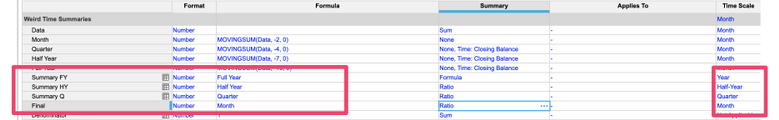Next, we need to set our summaries up.

For the ratio, we start with the highest level of the hierarchy, which in this case is the year. Since there is nothing higher than we can just make that be a formula since we are directly referencing it to our calculation line. From there, we move down to the Half Year, and we set the summary to Ratio being the level higher and dividing it by 1. To create the 1, I have a line called "denominator" that just has the formula of 1.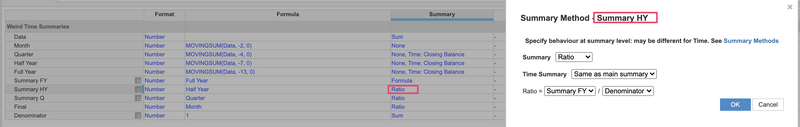Now do the same for the Summary Quarter Line with Ratio "Summary HY / Denominator"

Finally do the same for Month Summary (Final) and the Ratio Summary is "Summary Q / Denominator"

The result looks like this (Source of the data in Red, Final line in Green):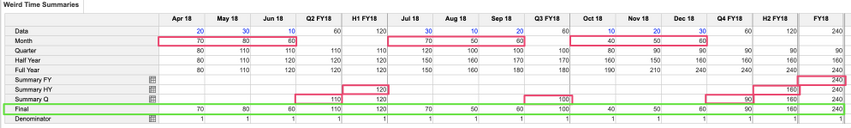Let me know if you have questions!

Jason

•Hi @CM ,

I don't think so we have a list that shows which functions support "Formula" in the summary method but let us know what are you trying to achieve?

Thanks

Akhtar

•Thanks Akhtar,

I have a requirement for a movingsum to work at different levels of the Time Hierarchy.

Months = Average the last 2 Months

Qtrs = Average the last 4 months

Half Yrs = Average the last 7 Months

Full Year = Average the last 13 Months

I have built a module using cTime (custom Time) and "cumulate" and it works - the issue is trying to get data into a module with the builtin time dimension using Months/QTR/Half/Full - It seems that any formula that uses "Lookup" to translate cTime to builtin Time, stops the summary method of "Formula" from working.

•Hi @CM

Create a mapping module from custom Time to Real-Time (Anaplan) and use the MovingSum() function on Real Time.  Then do a lookup to Real Time from the mapping module to get your data for reporting.

Hope this helps!

Thanks

Akhtar

•That wont work for two reasons:

A) When you use a "Lookup" you cant use "formula" as a summary method.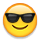MovingSum dosn't allow summary method of "formula", which is needed so that I can choose 4 periods at a QTR and 7 periods at half year etc.

Thanks Anyway.

•That will work perfectly!

I really appreciate the clear explanation and screenshots you've done as well!

Regards

Cam